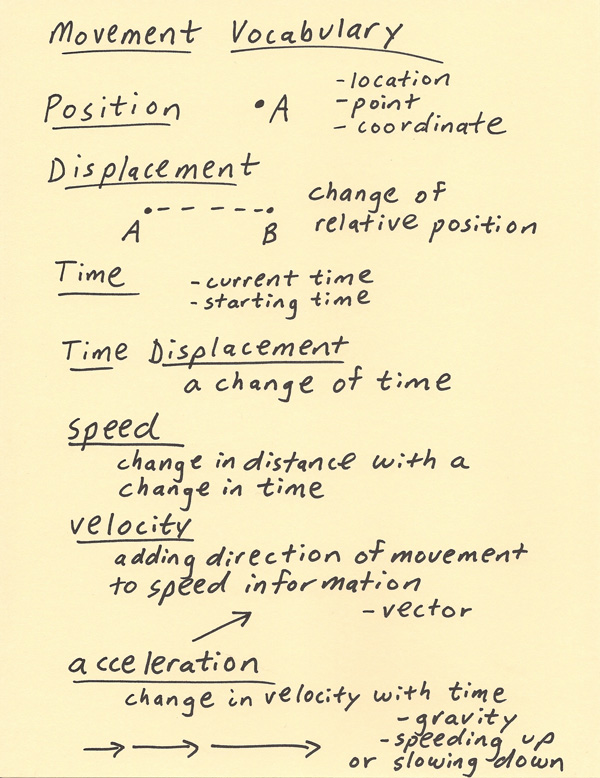[--MAIN HOME--] [--ALL HABYHINTS--] [--FACEBOOK PAGE--]

 HABYTIME MINI LECTURE 61: MOVEMENT VOCABULARY

METEOROLOGIST JEFF HABY

There are several terms that are used for position, motion and the type of motion. Many of these are explained below and a diagram summarizing these terms is shown on the bottom of the page:

Position: This is a stationary position relative to surrounding objects. In one dimension, position is noted as a point on a straight line. In two dimensions, position is notated with a coordinate. When graphing on a two dimensional surface such as with graph paper, position is indicated by an (x,y) coordinate. Treating Earth’s curved surface as the surface of a two dimensional sphere, position is indicated by using longitude and latitude. Position can also be indicated in three dimensional space (x,y,z).

Distance displacement: Displacement is the distance an object moves relative to where it was previously positioned. On a one dimensional surface, there is found from the difference between the starting and ending position along the line. On a two dimensional surface, it is the distance traveled from point A to point B on the surface. On a flat surface such as on graph paper, this distance can be solved by using the distance formula. There is also a distance formula for three dimensional space.

Time: Time is a dimension that flows in the direction from the past, to the current time to the future. Typically time is indicated by a relative current time, a starting time or a change in time. It is the change in time, or time interval, that is often needed in calculations. Negative or backward flowing time can be solved in equations, but it reality, time is always sensed as moving in the positive direction (toward the future).

Time Displacement: Time displacement is another term for a change in time which is found by the elapsed time between a starting and final time.

Speed: Speed is a change in distance (using distance units) with a change in time (using time units). Another way to say this is that it is a displacement in distance divided by a time displacement.

Velocity: The magnitude (with number value with units) is a speed but there is added information of direction. For example, 10 m/s is a speed while North at 10 m/s is a velocity. Velocity can also be described by using vectors on a coordinate grid.

Acceleration: Acceleration is a change in velocity with time. For example, 10 m/s per second is 10 m/s^2. Positive values indicate an object is increasing in speed (acceleration) toward a particular direction. Negative values indicate an acceleration in the opposite direction from what is labeled as positive on the coordinate axis. For example, vertically up is often labeled as the positive direction, and since gravity is toward the surface then gravity will be indicated as a negative g (-g). A negative acceleration that occurs in the direction labeled as positive indicates an object is decelerating or slowing down relative to the positive direction it is moving toward. In other words, it is accelerating in the opposite direction in order to slow down in the positive direction.